Strain Energy Density Plot PropertyManager

The Strain Energy Density Plot PropertyManager allows you to plot strain energy per unit volume after running a harmonic or random vibration study.

Display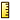Units Select a unit for the strain energy density component.

 Show PSD value Available for random vibration studies only. If checked, the PSD of the strain energy density is displayed at a particular solution step. Otherwise, the RMS of the strain energy density for the solution range is displayed. Element values Generates elemental strain plots (one value/color for each element).

Plot Step

For random vibration studies, available if Show PSD value is checked.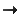Plot for Single Step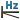Displays the frequency corresponding to the selected plot step. Plot Step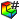Sets the step number at which the selected result is to be plotted.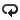Plot Bounds across All Steps
 Maximum Displays the maximum algebraic values (envelope plot) across all solution steps. Minimum Displays the minimum algebraic values (envelope plot) across all solution steps.

Deformed Shape

If checked, the strain energy density component is plotted on the deformed shape of the model.

 Automatic Displays the default Scale Factor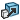that the program uses to scale the largest deformation to 10% of the largest dimension of the smallest box that surrounds the model. True scale Displays the actual deformed shape of the model (Scale Factor is 1.0). User defined Allows you to enter your own Scale Factor. In most cases, a larger scale factor helps to appreciate the deflection.

Property

 Include title text If checked, type a custom title for the plot. Associate plot with name view orientation Allows you to associate a predefined view orientation with the active plot.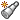Associate with current view Allows you to associate the current view with the active plot.